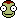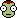• 0

# How do you calc deltaV per fuel unit on an engine?

## Question

How do you calculate deltaV per fuel unit on an engine?

Say ive got engine X with 60 units of fuel (or oxidizer, if its easier). How much delta V can I get out of that?

## Recommended Posts

• 0

The short answer is: You don't.

Delta-v is dependent on the efficiency of the engine(s) and the ratio of the vehicle's total mass to its dry mass (i.e. final mass after all the fuel is burned up). Delta-v is essentially a measure of the total amount a rocket can accelerate; in a perfect, featureless vacuum, a rocket with 1000 m/s of delta-v can accelerate from a standstill to a velocity of 1000 m/s, or it can accelerate to 500 m/s, then turn around and decelerate back to a standstill (or any combination of maneuvers that adds up to 1000 m/s of velocity).

The way you can calculate delta-v directly is actually pretty simple:

delta_v = Isp * 9.8 m/s2 * LN(initial_mass / dry_mass)

This is also known as Tsiolkovsky's rocket equation (or just "the rocket equation"), and can easily be done on the calculator that ships with your computer or with a pocket calculator that has an "LN" key (i.e. that can calculate the natural logarithm of a given number).

Whenever the subject comes up, I tend to recommend MyKSPCareer.com and these three pages on Atomic Rockets if you want a more detailed explanation. The Atomic Rockets pages in particular helped me out considerably when I was trying to wrap my head around the concept when I started playing.

Hope this helpsEdited by Specialist290
Almost forgot the most important part!
##### Share on other sites

• 0

It wouldn't be hard to do, you just have to know how much that bit of fuel weighs. Since the game measures weight in tonnes, we'll stick to those units. One unit of fuel weighs 0.005 tonnes. If you are using an LV-N as your engine, and the craft has a mass of, let's say 20 tonnes. Burning 60 units of fuel causes your mass to decrease by 0.3 tonnes. Plug this into the rocket equation, Isp*9.81*ln(mass 1/mass 2), and you get 118.6 m/s of delta v. The thing you have to remember is that units of fuel needs to be fuel+oxidizer, not just the fuel.

Also, since starting mass is different, you can't find a "deltaV per unit of fuel" as each unit has slightly more than the last burned, because total mass is decreasing. You can only calculate deltaV in chunks of mass.

Edited by Specialist290
Merging sequential posts.
##### Share on other sites

• 0

As stated before, you have to use the Tsiolkovsky rocket equation, but the amount of DV per liter of fuel is not constant. If you burn a 1 t of fuel when you mass at 100 t, that fuel has to push the rest of the ship's mass, including fuel. That means if you burn 1 t of fuel on the same ship at a mass of 50 t, that fuel will provide considerably more DV than the first ton because it is not being used to push as much mass.

##### Share on other sites

• 0

Easy way:

9.81*Isp * ln { current weight / ( current weight - 20/11 * oxidizer*5/1000) if oxidizer is limiting factor. else replace that term with 20/9 * liquidfuel * 5/1000

##### Share on other sites

• 0

This is why engine efficiency is measure in ISP.

##### Share on other sites

• 0

Moreover, the dV you get from the first "unit" of fuel will be different for the dV you get from the last "unit" of fuel, because the mass ratio changes. That makes any attempt to define dV in terms of fuel units a poor estimation at best.

=Smidge=

##### Share on other sites

• 0
Moreover, the dV you get from the first "unit" of fuel will be different for the dV you get from the last "unit" of fuel, because the mass ratio changes. That makes any attempt to define dV in terms of fuel units a poor estimation at best.

=Smidge=

Actually - that's inaccurate. You can calculate the Dv for any arbitrary portion of your fuel so long as you plug the numbers into the equation correctly.

That is, given delta_v = Isp * 9.8 m/s2 * LN(initial_mass / dry_mass)

=> delta_v = Isp * 9.8 m/s2 * LN(initial_mass / remaining_mass) + Isp * 9.8 m/s2 * LN(remaining_mass / dry_mass)

So you can very accurately calculate total delta-v at for any sub-quantity of fuel. This is also how you can use this single equation to calculate total delta-v for your rocket, given multiple states - you just calculate the Dv for each stage, and add it together. You are quite correct in one thing - the delta-v for the first half and delta-v for the second half will be quite different, assuming you spent 50% of your propellant in each stage, because the empty mass is different.

##### Share on other sites

• 0

You've seen that calculations are dependent on several variables which constantly change. An alternative might be to use a mod such as Kerbal Engineer to use in tuning your design. During flight, with the Vessel tab selected, you can see some variables (mass, dV, TWR) change in real time. This can further help you tune your design.

##### Share on other sites

• 0

Wow, so many ways to disagree and yet say the same thing...

You CAN calculate the dV for a given unit of fuel, but the dV for a given unit of fuel is not a constant.

So maybe we should ask the OP what he/she actually wants to know?

##### Share on other sites

• 0
Wow, so many ways to disagree and yet say the same thing...

You CAN calculate the dV for a given unit of fuel, but the dV for a given unit of fuel is not a constant.

Yes, because you have to account for the whole system. It is not miles per gallon in any sense of the word. it is miles per gallon per weight per engine power, more like. All must be figured in.

##### Share on other sites

• 0

At some point, I made an spread sheet calculating delta-V over time. Knowing the fuel consumption rate, I could calculate the total amount of fuel used at any time after the engine is activated. Subtract that from initial mass, and plug it into the rocket equation.

Just do the same thing but cut out the middleman of time. Make a spread sheet with units of propellant used on one column, and calculate out delta-V in another based on the rocket equation.

So for a LV-N rocket (vacuum Isp 800 s), with an initial mass of 100t, and fuel mass of 0.005t per propellant unit, something like this: "=800*9.81*ln((100-(0.005*X)))" where X is the cell with units used.

##### Share on other sites

• 0
Yes, because you have to account for the whole system. It is not miles per gallon in any sense of the word. it is miles per gallon per weight per engine power, more like. All must be figured in.

Yes, that was my point. In addition to the point that we all have different ways of saying exactly the same thing.

Maybe another way of saying my point is that we haven't asked the OP what he/she is really looking for. We just keep restating the rocket equation over and over with words, numbers, and examples.

##### Share on other sites

• 0
Maybe another way of saying my point is that we haven't asked the OP what he/she is really looking for. We just keep restating the rocket equation over and over with words, numbers, and examples.

From my perspective, the OP wanted to know if you can calculate a certain deltaV per unit of fuel, and then multiply it by the units of fuel to get deltaV.

But then he/she also asked if it was possible to calculate deltaV, given an amount of 60 units of fuel. While the answer to the first question is no, the answer to the second is yes. With all the answers I'm sure he/she will find something satisfactory.

##### Share on other sites

• 0

Ok, I see what you're asking. Your asking if X engine provides 100 m/s dV per 1 unit of fuel, then 10 units provides 1 km/s dV. It does not work like that. There is a whats called "The Tyranny of Rockets Equation". Essentially, The amount of dV available does not follow a linear path depending on the amount of fuel you have.

Lets go to the VAB. I use Mechjeb to calculate dV, and if I take a Stayputnik, 1 Toroidal Fuel Tank, attach 2 small, fixed solar panels and a Rockomax 48-7S, this gives my probe 1,612 m/s of dV. So in theory adding another tank should give me 3,224 m/s of dV, right? nope. A second yields a total of 2,474 m/s of dV. Just half of our original value. So adding a third nets us how much? Not even 600 m/s more. Keep adding and you'll continue to get diminishing results, eventually to the point where adding another tank would provide another measly 10 m/s of dV.

##### Share on other sites

• 0

i agree with all of those, but opinions vary.

##### Share on other sites

• 0
3 hours ago, Sweetnsaltyish said:

i agree with all of those, but opinions vary.

In science there are no opinions that matter.

##### Share on other sites

• 0

Opinions especially don't matter when the original question was asked and answered over five years ago, and everyone involved has presumably long since moved on.Accordingly, locking thread to prevent further confusion. Anyone who has their own questions about dV (or anything else, for that matter) is welcome to start a new thread.